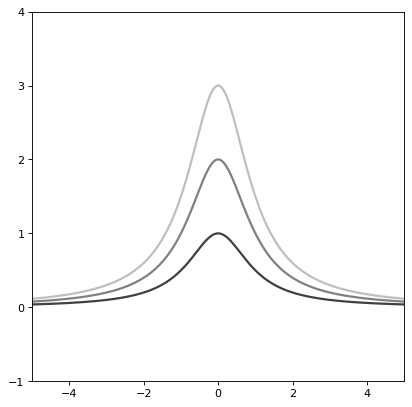# Moffat1D公司¶

class astropy.modeling.functional_models.Moffat1D(amplitude=1, x_0=0, gamma=1, alpha=1, **kwargs)[源代码]

amplitude浮动

x_0浮动

Moffat模型最大值的x位置。

gamma浮动

Moffat模型的核心宽度。

alpha浮动

fixed口述，可选

tied可选的

bounds可选的

eqcons可选列表

ineqcons可选列表

$f（x）=A\左（1+\frac{\left（x-x{0}\right）^{2}}{\gamma^{2}}\right）^{-\alpha}$

import numpy as np
import matplotlib.pyplot as plt

from astropy.modeling.models import Moffat1D

plt.figure()
s1 = Moffat1D()
r = np.arange(-5, 5, .01)

for factor in range(1, 4):
s1.amplitude = factor
s1.width = factor
plt.plot(r, s1(r), color=str(0.25 * factor), lw=2)

plt.axis([-5, 5, -1, 4])
plt.show()


(png _, svgpdf莫法特全宽半最大。 此属性用于指示evaluate方法所需的单元或单元集，并返回将输入映射到单元（或 None 如果接受任何单位）。 描述此类型模型的参数的名称。

 evaluate(x, amplitude, x_0, gamma, alpha) 一维Moffat模型函数 fit_deriv(x, amplitude, x_0, gamma, alpha) 一维Moffat模型参数导数

alpha = Parameter('alpha', value=1.0)
amplitude = Parameter('amplitude', value=1.0)
fwhm

gamma = Parameter('gamma', value=1.0)
input_units
param_names = ('amplitude', 'x_0', 'gamma', 'alpha')

x_0 = Parameter('x_0', value=0.0)

static evaluate(x, amplitude, x_0, gamma, alpha)[源代码]

static fit_deriv(x, amplitude, x_0, gamma, alpha)[源代码]